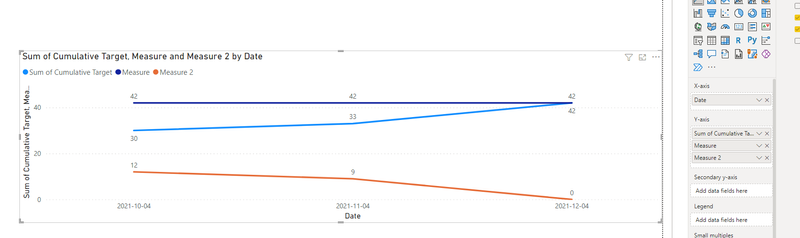cancel
Showing results for
Did you mean:Frequent Visitor

## Set value on last date for all rows

I have a set of data that looks similar to this:

 Date Type Cumulative Target Delivered 10/04/2021 Pipe 30 30 11/04/2021 Pipe 33 32 12/04/2021 Pipe 42 41

I'm trying to show this data in a line graph where I want the overall total target for the end of the year as a straight line, like a target line. I'm also going to use the total target to subtract the current delivered at each date to have a burndown of how many are left.

i.e. at the latest date (12/04/2021) the cumulative target is 42, so I want 42 to be a constant straight line across the graph. However I can't get it to display without having it break down into each date. I've created a measure using:

CALCULATE(MAX(Table[Cumulative Target]), Table[Date] = DATE(YEAR(TODAY()),12,31))

But this is 0 for all dates and just gives me a dot at the end of the year with the total targeted. I can't use this for a target line or to subtract the deliveries from etc.

Is there a way to have my measure just calcualate the value at that date and then store that value as a variable, not tied to any date? Or can I add a new column to my table somehow that shows the max on every row for subtraction? e.g.

 Date Type Cumulative Target Delivered Max Target 10/04/2021 Pipe 30 30 42 11/04/2021 Pipe 33 32 42 12/04/2021 Pipe 42 41 42

1 ACCEPTED SOLUTIONSuper User

Max Target will be

=

var _max = maxx(allselected(Table), Table[Date])

return

calculate(Max(Table[Cumulative Target]), Filter(allselected(Table) , Table[Date] = _max))

3 REPLIES 3Super User

Hi,

If you a certain type, the figures in the cumulative type column are always in ascending order (as sorted by Date), then this measure will work

Measure 1 = calculate(max(Data[cumulative type]),all(Calendar[Date]))

My assumption is that cumulative type is a column in the Data Table (and not a measure).

Ensure that there is a Calendar Table.  There should be a relationship between the Date column of the Data Table to the Date column of the Calendar Table.  To your visual, drag Date from the Calendar Table.

Hope this helps.

Regards,
Ashish Mathur
http://www.ashishmathur.comCommunity Support

Hi  @SClarke501 ,

Here are the steps you can follow：

1. Create measure.

``````Measure =
MAXX(ALL('Table'),[Cumulative Target])``````
``````Measure 2 =
[Measure] - MAX('Table'[Cumulative Target])``````

2. Result:If this method does not meet your needs, you can provide us with your special sample data and the desired output sample data in the form of tables, so that we can better help you solve the problem.

Best Regards,

Liu Yang

If this post helps, then please consider Accept it as the solution to help the other members find it more quicklySuper User

Max Target will be

=

var _max = maxx(allselected(Table), Table[Date])

return

calculate(Max(Table[Cumulative Target]), Filter(allselected(Table) , Table[Date] = _max))